Friday , May 24 2019
Home » Igcse Physics Revision Notes » Work Energy And Power

# Work Energy And Power

### Work:

Work done by a constant force is given by the product of the force and the distance moved in the direction of the force.

Work = Force (N) × Distance (m)

The SI unit for work is Joule (J).

Remember:

Work is not done when

• The direction of the applied force and the direction in which the object moves are perpendicular to one another.
• The force is applied on the object (such as the wall) but the object doesn’t move.

### Energy:

Energy can be defined as the ability to do work.

SI unit: Joule (J)

### Types Of Energy: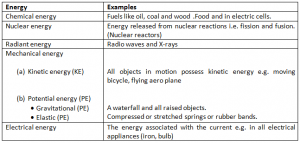### Kinetic Energy:

It is the energy possessed by a body by virtue of its motion .In simpler words ,any moving object has kinetic energy.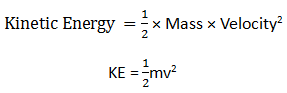SI unit: Joules (J)

### Gravitational Potential Energy:

Gravitational potential energy = Mass × Gravity ×Height

GPE =mgh

### Kinetic And Potential Energy In Simple Pendulum: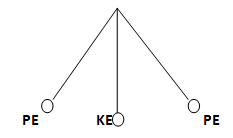### Conversion And Conservation Of Energy:

Energy can neither be created nor destroyed in any process. It can be converted from one form to another or transferred from one body to another, but the total amount remains constant.

### Power:

Power is defined as the rate of work done or energy converted.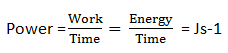SI unit for power is Watts (w)

Therefore work =power × time

Energy =power × time

### Efficiency Of A Machine:

Efficiency of a machine is a ratio of output work to input work.

It shows the percentage of output work from the input work.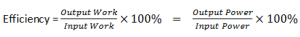No machine is hundred percent efficient because there is always energy in the form of  heat and sound.

### About Admin## Mass Weight And Density

Mass: Mass is the measure of amount of substance in a body. (Irrespective of its …